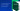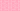Pricing# What is regression analysis?

Performing a basic regression analysis is a simple way to compare two or more pieces of data. In business, this type of analysis is used to calculate cause and effect or even to forecast future trends. Learn more about how to run a regression analysis below.

## Understanding regression analysis

The regression analysis method compares two (or more) sets of variables, where one is dependent on the other. It determines the relationship between the pair but can also indicate that there is no existing relationship. For example, a business might run a regression analysis on its various advertising forms, comparing advertising with sales figures. The analysis might show that money spent on online advertising has increased sales, yet mailed flyers have had no impact.

## Regression analysis variables

To get started with understanding regression analysis, you must first look at the variables involved. A regression model weighs up the relationship between two variables, one of which is dependent on the other. If we take the relationship between online advertising costs and sales figures mentioned above, the advertising costs would be the independent variable, and the sales figure the dependent variable. This is because the sales figures go up and down depending on your advertising spend.

### Covariance

To forecast how much these sales figures will fluctuate, you must figure out the strength of the relationship between the dependent and independent variables. This relationship is called the covariance. If both variables increase, the covariance is positive. If one goes down when the other goes up, the covariance is negative.

### Correlation

Another factor involved in basic regression analysis is the correlation between the two variables. To calculate a correlation, you take the covariance and divide it by the standard deviation added together between the two variables. This results in a figure between -1 and +1.

## How to run a regression analysis

### Step 1: Form a hypothesis

We’ve touched on variables and their relationship above. To run a regression analysis, you’ll need to select the two variables that you believe are related. Form your hypothesis about their relationship, gathering as much data as possible. In the case of the advertising and sales relationship, it’s better to collect financial data over the course of a year for a more accurate analysis.

### Step 2: Create a chart

The next step is to chart your data. With two sets of data, you can create a basic line chart for linear regression. One variable will be charted on the X-axis, and the other on the Y-axis. Entered in a spreadsheet, you’ll then be able to see the correlation between the variables. If there’s a straight line, this shows a positive correlation.

### Step 3: Analyse the results

By examining the chart in a basic linear regression, you’ll be able to see the intercept, coefficient, and correlation. Taking these figures together shows the historical relationship between two data sets, allowing you to forecast how the model will look in the future. If historically, there is a positive relationship between online advertising and sales, you can plug the percentages into a model to generate a forecast for next year. This can help with budgeting for the upcoming financial year, though there are some limitations of regression analysis when no relationship can be determined between certain variables.

## Further applications of regression analysis

In finance, regression analysis is used to calculate the Capital Asset Pricing Model (CAPM), which determines the relationship between market risk premium and expected return. It’s also used to forecast securities returns or future performance in business. The simple slope function in spreadsheet accounting programmes can chart a stock’s volatility of returns, using regression analysis as its basis.

Finally, regression analysis helps forecast a company’s financial statements. By investigating the relationships between different variables or business drivers, you can predict revenues and expenses more accurately.

## We can help

GoCardless helps you automate payment collection, cutting down on the amount of admin your team needs to deal with when chasing invoices. Find out how GoCardless can help you with ad hoc payments or recurring payments.

GoCardless makes it easy to collect recurring payments